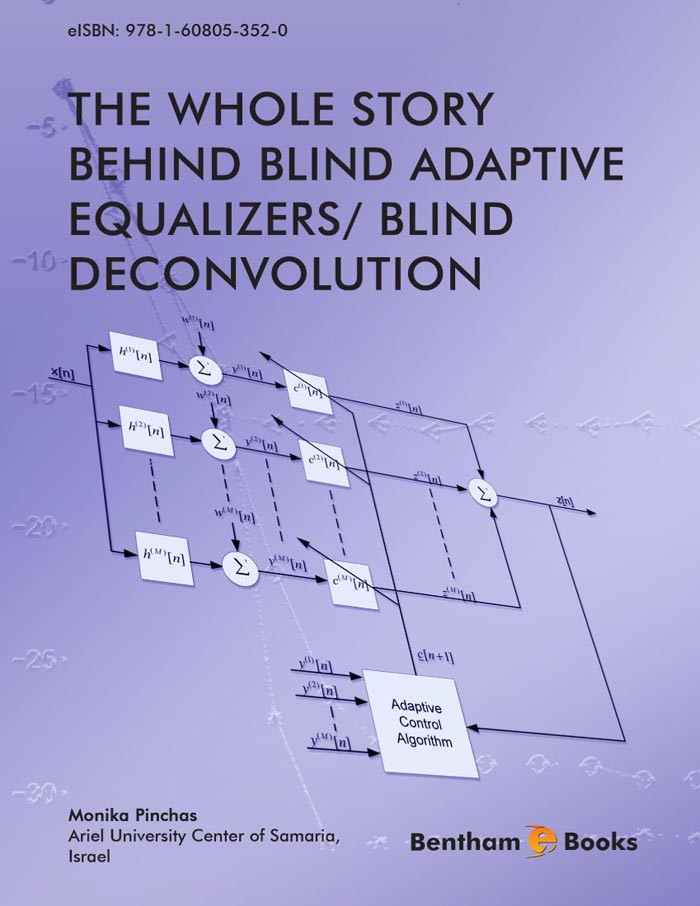### The Bayesian Approach

#### Abstract

In this chapter the Bayesian approach for blind deconvolution is explained. We cover the dierent proposed expressions for the conditional expectation known in the literature and explain in detail the advantages and disadvantages of each ob- tained expression. &lt;/P&gt; &lt;P&gt; In the literature, the convolutional noise probability density function (pdf) is modeled as a Gaussian pdf. In this chapter, a new closed-form approximated ex- pression is derived for the conditional expectation based on a new model for the convolutional noise pdf. The new derived expression for the conditional expecta- tion, is based on the Maximum Entropy approach, Edgeworth expansion, Laplace integral method and is valid for the noiseless, real valued and two independent quadrature carrier case. According to simulation results carried out for signal to noise ratio (SNR) SNR = 30 [dB], the new derived expression leads to improved equalization performance for the 16QAM input constellation case.

Total Pages: 43-67 (25)